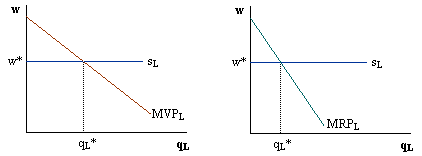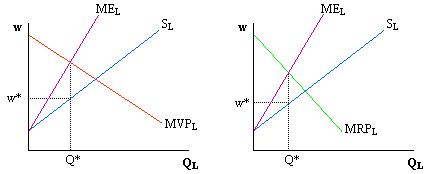Barry Haworth
University of Louisville
Department of Economics
Economics 301

### Different Factor Markets

In the short run, let's assume that labor is the only variable factor hired by different firms. Equilibrium in the market depends on two things. First, we must know whether the firms buying labor are competitive or monopolistic in their respective output markets. Second, we must know whether the labor market itself is competitive or monopsonistic. These different situations are illustrated below.

Assume that the labor market is competitive, so that firms hire where demand equals supply. In this situation, firms face a horizontal labor supply curve. If the firm is perfectly competitive in its output market, then its labor demand curve is called the marginal value product (MVP). If the firm is a monopolist in its output market, then its labor demand curve is called the marginal revenue product (MRP).The graph (above) on the left represents the perfectly competitive firm buying labor from this competitive labor market, whereas the graph on the right represents the monopolistic firm buying labor from this competitive labor market. In each case, labor is hired and paid a wage that corresponds with the point where the demand for labor equals the supply of labor.

If the labor market involves monopsony, then firms hire where their marginal expenditure curve crosses their demand for labor curve and pay a wage that corresponds with the labor supply curve. Depending on whether the firm is perfectly competitive in its output market or monopolistic, the firm's demand for labor is either called the marginal value product curve (left graph below) or the marginal revenue product curve (right graph below).In both situations, and in the absence of any unionization, the wage is determined by where the vertical dotted line (coming down from the intersection of either MVPL and MEL to Q* on the left graph or from MRPL and MEL to Q* on the right graph) crosses the labor supply curve. The wage in each case is given as w*.